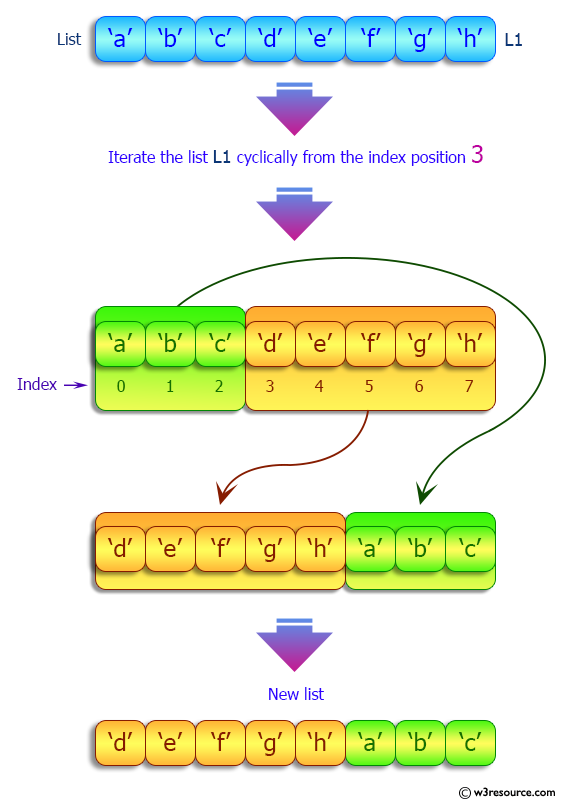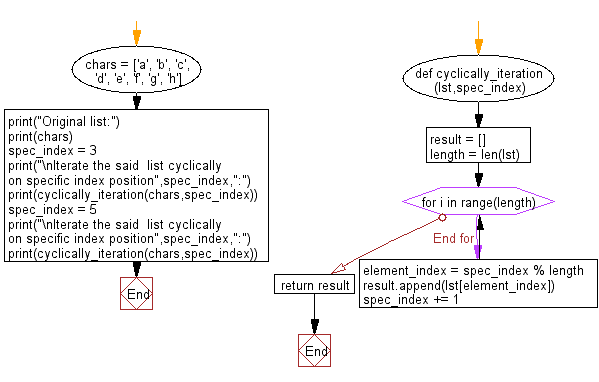﻿ Python: Iterate a given list cyclically on specific index position - w3resource# Python: Iterate a given list cyclically on specific index position

## Python List: Exercise - 181 with Solution

Write a Python program to iterate a given list cyclically on specific index position.

Sample Solution:

Python Code:

``````def cyclically_iteration(lst,spec_index):
result = []
length = len(lst)
for i in range(length):
element_index = spec_index % length
result.append(lst[element_index])
spec_index += 1
return result

chars = ['a', 'b', 'c', 'd', 'e', 'f', 'g', 'h']
print("Original list:")
print(chars)
spec_index = 3
print("\nIterate the said  list cyclically on specific index position",spec_index,":")
print(cyclically_iteration(chars,spec_index))
spec_index = 5
print("\nIterate the said  list cyclically on specific index position",spec_index,":")
print(cyclically_iteration(chars,spec_index))
```
```

Sample Output:

```Original list:
['a', 'b', 'c', 'd', 'e', 'f', 'g', 'h']

Iterate the said  list cyclically on specific index position 3 :
['d', 'e', 'f', 'g', 'h', 'a', 'b', 'c']

Iterate the said  list cyclically on specific index position 5 :
['f', 'g', 'h', 'a', 'b', 'c', 'd', 'e']
```

Pictorial Presentation:Flowchart:## Visualize Python code execution:

The following tool visualize what the computer is doing step-by-step as it executes the said program:

Python Code Editor:

Have another way to solve this solution? Contribute your code (and comments) through Disqus.

What is the difficulty level of this exercise?

Test your Python skills with w3resource's quiz

﻿

## Python: Tips of the Day

```print(2_000_000)
```2000000